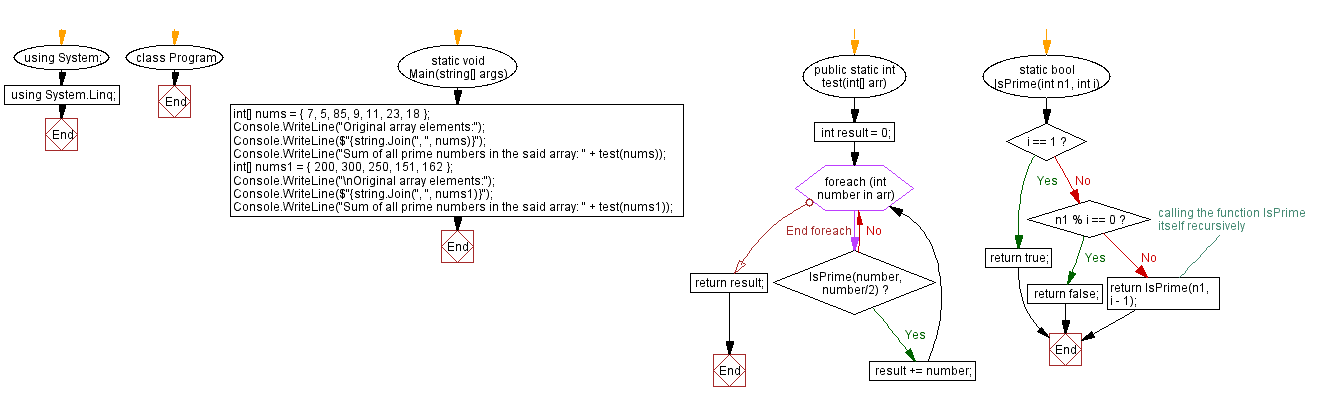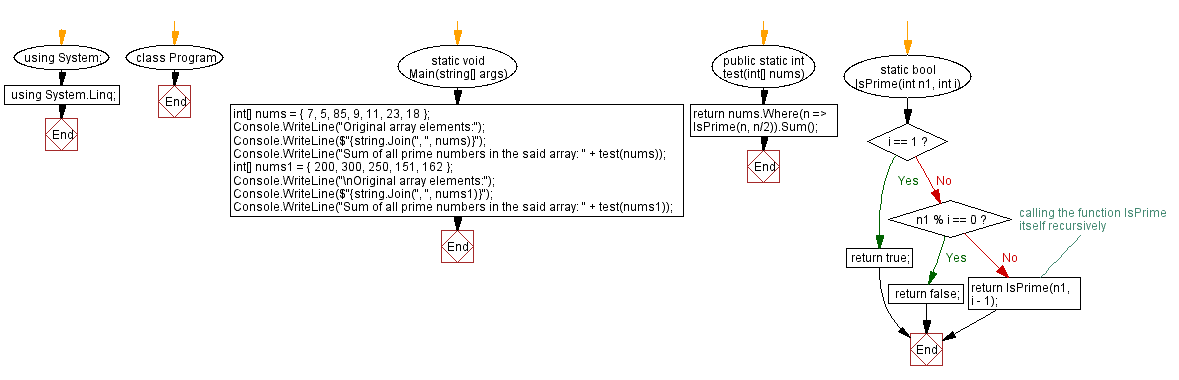﻿ C# - Sum of all prime numbers in an array# C# Sharp Exercises: Sum of all prime numbers in an array

## C# Sharp Array: Exercise-39 with Solution

Write a C# Sharp program that calculates the sum of all prime numbers in an array of numbers.

Sample Data:
({ 7, 5, 85, 9, 11, 23, 18 }) -> 46
({ 200, 300, 250, 151, 162 }) -> 151

Sample Solution-1:

C# Sharp Code:

``````using System;
using System.Linq;
namespace exercises
{
class Program
{
static void Main(string[] args)
{
int[] nums = { 7, 5, 85, 9, 11, 23, 18 };
Console.WriteLine("Original array elements:");
Console.WriteLine(\$"{string.Join(", ", nums)}");
Console.WriteLine("Sum of all prime numbers in the said array: " + test(nums));
int[] nums1 = { 200, 300, 250, 151, 162 };
Console.WriteLine("\nOriginal array elements:");
Console.WriteLine(\$"{string.Join(", ", nums1)}");
Console.WriteLine("Sum of all prime numbers in the said array: " + test(nums1));
}
public static int test(int[] arr)
{
int result = 0;
foreach (int number in arr)
{
if (IsPrime(number, number/2))
{
result += number;
}
}
return result;
}

static bool IsPrime(int n1, int i)
{
if (i == 1)
{
return true;
}
else
{
if (n1 % i == 0)
return false;
else
return IsPrime(n1, i - 1);//calling the function IsPrime itself recursively
}
}
}
}
```
```

Sample Output:

```Original array elements:
7, 5, 85, 9, 11, 23, 18
Sum of all prime numbers in the said array: 46

Original array elements:
200, 300, 250, 151, 162
Sum of all prime numbers in the said array: 151

```

Flowchart:Sample Solution-2:

C# Sharp Code:

``````using System;
using System.Linq;
namespace exercises
{
class Program
{
static void Main(string[] args)
{
int[] nums = { 7, 5, 85, 9, 11, 23, 18 };
Console.WriteLine("Original array elements:");
Console.WriteLine(\$"{string.Join(", ", nums)}");
Console.WriteLine("Sum of all prime numbers in the said array: " + test(nums));
int[] nums1 = { 200, 300, 250, 151, 162 };
Console.WriteLine("\nOriginal array elements:");
Console.WriteLine(\$"{string.Join(", ", nums1)}");
Console.WriteLine("Sum of all prime numbers in the said array: " + test(nums1));
}
public static int test(int[] nums)
{
return nums.Where(n => IsPrime(n, n/2)).Sum();
}

static bool IsPrime(int n1, int i)
{
if (i == 1)
{
return true;
}
else
{
if (n1 % i == 0)
return false;
else
return IsPrime(n1, i - 1);//calling the function IsPrime itself recursively
}
}

}
}

```
```

Sample Output:

```Original array elements:
7, 5, 85, 9, 11, 23, 18
Sum of all prime numbers in the said array: 46

Original array elements:
200, 300, 250, 151, 162
Sum of all prime numbers in the said array: 151
```

Flowchart:C# Sharp Code Editor:

Contribute your code and comments through Disqus.

Previous C# Sharp Exercise: Check specific digit in an array of numbers.
Next C# Sharp Exercise: Smallest positive which is not present in an array.

What is the difficulty level of this exercise?

Test your Programming skills with w3resource's quiz.

﻿